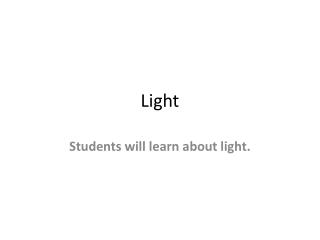DownloadDownload PresentationLight

# Light

Download Presentation## Light

- - - - - - - - - - - - - - - - - - - - - - - - - - - E N D - - - - - - - - - - - - - - - - - - - - - - - - - - -
##### Presentation Transcript

1. Light Students will learn about light.

2. Light • Light is a transverse electromagnetic wave. Consider the electric field portion as transverse up and down and the magnetic field portion as perpendicular side to side. • Light has many wave properties: reflection, refraction, diffraction, interference patterns, Doppler effect, color dependent on frequency, and intensity (or brightness) dependent on the amplitude of the waves.

3. Speed of light • The speed of light in a vacuum (and also in air) is 3 x 10^8 m/s. • Albert Einstein showed that nothing, nowhere, no how can ever every go faster than the speed of light = 3 x 10^8 m/s.

4. Wave Theory of Light • Wave Theory supported by observations that light exhibits diffraction (through one slit approximately one wavelength) and interference (Young’s double-slit experiments) • Wave Theory explains refraction of light and the fact that light travels more slowly in denser media

5. Light as a particle • Sometimes light acts as a particle • Light “particles” are called “photons” • Light colliding with a mirror makes very small “pings” • Photoelectric Effect: Light delivers energy through its frequency in order to excite and give electrons kinetic energy. Light energy (as photons): E = hf • H = 6.63 x 10^-34 Joule seconds

6. Photoelectric Experiment (1887 Hertz; 1905 Planck; Einstein; 1913 Millikan) • Vibrational energy is “quantized” or “discrete” (example: piano or flute or staircase with steps; not smooth or continuous) • Light is transmitted as tiny particles or “photons” (the most basic quantum of light energy). • Photoelectric effect is when light shining on metal causes electrons to be emitted from the surface with various kinetic energies

7. Wave Theory Predictions • 1. Red light does not cause electrons to be emitted. So, let us increase the intensity of the red light, then the number of electrons and their kinetic energy should be increased. • 2. Frequency should not affect the kinetic energy of the ejected electrons.

8. Photon Theory • 1. An increase in intensity of the light means more photons so more electrons will be ejected, but the kinetic energy of the electrons will not be changed. • 2. If the frequency of the light is increased (example: from red to blue light) the maximum kinetic energy of the electrons increases linearly: E = hf • 3. There exists a minimal cutoff frequency where no electrons would be emitted.

9. Electromagnetic Spectrum Gamma rays 10^19 – 10^21 Hertz 10^-12 m • X rays 10^17 – 10^20 Hertz 10^-10 m • Ultraviolet 10^15 – 10^18 Hertz 10^-7 m • Visible 10^14 – 10^15 Hertz 10^-6 m • Infrared 10^12 – 10^14 Hertz 10^-5 m • Microwave 10^9 – 10^12 Hertz .01 m • FM /TV 10^8 Hertz 3 m • AM 10^6 Hertz 200 m

10. Dispersion • Dispersion is the spreading of white light into the full spectrum. A prism separates white light into a rainbow of colors. This happens because the index of refraction of the material depends on the wavelength. Different wavelengths are bent to varying degrees. • Violet is bent the most; red is bent the least. • The sky is blue because it is bent the most as sunlight comes through earth’s atmosphere.

11. Examples of Dispersion • Prism • Rainbow • Diamonds

12. Polarized light • Ordinary light is unpolarized. It has many vibrations in many planes at once. The electric field vectors vibrate at all angles. • Light can be “filtered” or “polarized” by passing it through parallel slits. Long molecules can be arranged as long slits to achieve this purpose. • Example: polarizing lenses in Polaroid sunglasses filter out “glare”